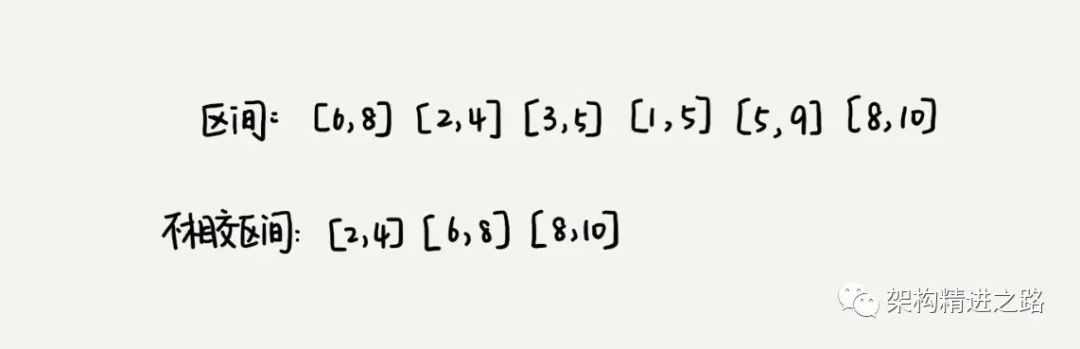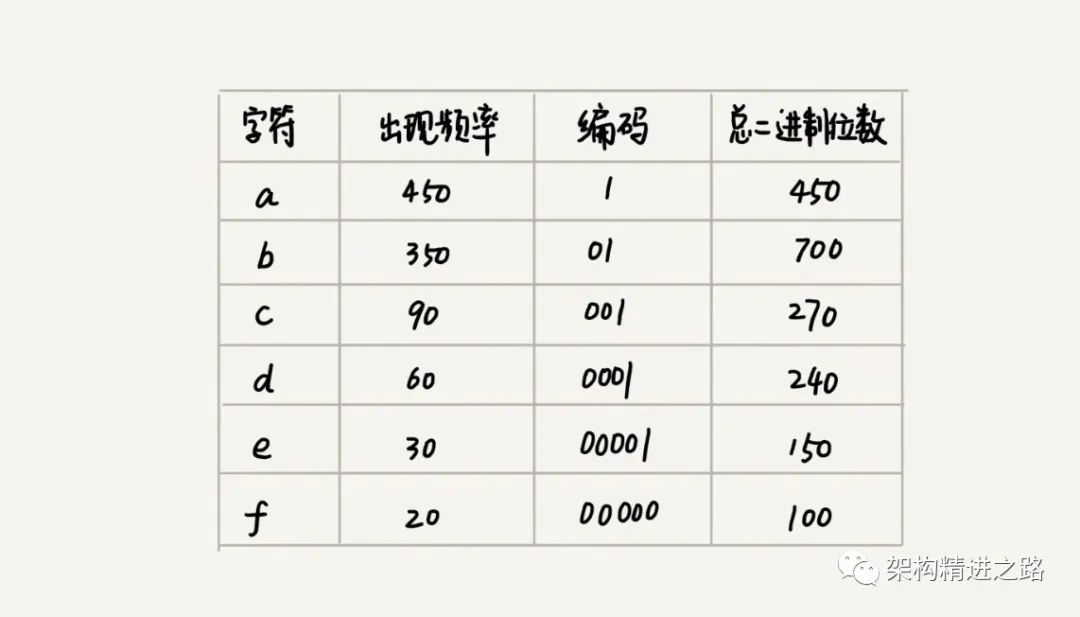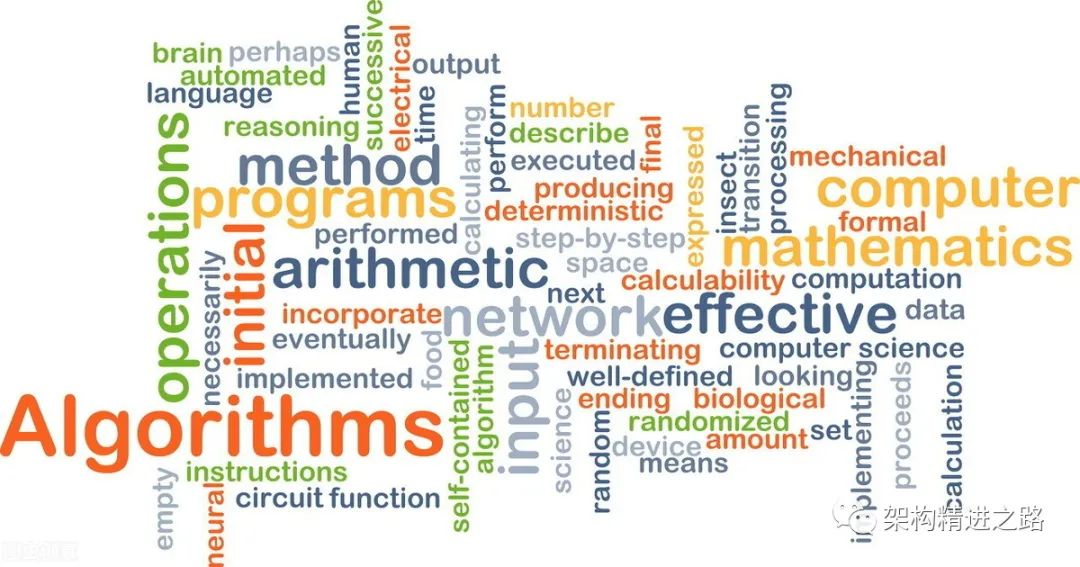# 面试常见的四种算法思想，全在这里了我是架构精进之路，点击上方“关注”，坚持每天为你分享技术干货，私信我回复“01”，送你一份程序员成长进阶大礼包。

# 例子3# 用贪心算法实现霍夫曼编码

a(000)、b(001)、c(010)、d(011)、e(100)、f(101)# 2、分治

• 分解：将原问题分解成一系列子问题；

• 解决：递归地求解各个子问题，若子问题足够小，则直接求解；

• 合并：将子问题的结果合并成原问题。

• 原问题与分解成的小问题具有相同的模式；

• 原问题分解成的子问题可以独立求解，子问题之间没有相关性，这一点是分治算法跟动态规划的明显区别，等我们讲到动态规划的时候，会详细对比这两种算法；

• 具有分解终止条件，也就是说，当问题足够小时，可以直接求解；

• 可以将子问题合并成原问题，而这个合并操作的复杂度不能太高，否则就起不到减小算法总体复杂度的效果了。

# 分治算法应用举例分析

1. 假设有n个数据，期望数据从小到大排序，那完全有序的数据的有序度就是n(n-1)/2。逆序度等于0；相反，倒序排序的数据的有序度就是0，逆序度是n(n-1)/2。除了这两种极端情况外，我们通过计算有序对或逆序对的个数，来表示数据的有序度或逆序度。

2. 现在问：如何编程求出数组中的数据有序对个数或逆序对个数？

3. 最简单的办法：拿每个数字和他后面的数字比较，看有几个比它小。将比它小的数字个数记作k，通过这样的方式，把每个数字都考察一遍后，对每个数字对应的k值求和，最后得到的总和就是逆序对个数。但时间复杂度是O(n^2)。

4. 用分治算法，套用分治的思想，将书中分成前后两半A1和A2，分别两者中的逆序对数，然后在计算A1和A2之间的逆序对个数k3。那整个数组的逆序对个数就是k1+k2+k3。

5. 要快速计算出两个子问题A1和A2之间的逆序对个数需要借助归并排序算法。

# 求两个数的最大共因子（欧几里得算法）

<?php

/**
* 分治算法
* 逻辑：
* (1) 找出基线条件，这种条件必须尽可能简单。
* (2) 不断将问题分解（或者说缩小规模），直到符合基线条件
*/
class dc {
/**
* 最大公因子（欧几里得算法）
* 可以引申到-客厅长宽固定，问选择多大的正方形地砖，可以正好铺满客厅
* @param $a * @param$b
* @return mixed
*/
function greatestCommonFactor($a,$b) {
if ($a <$b) {
$c =$a;
$a =$b;
$b =$c;
}
$c =$a % $b; if ($c == 0) {
return $b; } else {$n = $this->greatestCommonFactor($c, $b); } return$n;
}
}

dd((new dc())->greatestCommonFactor(160, 56));


# 分治思想在海量数据处理中的应用

1. 假设，给10GB的订单文件按照金额排序这样一个需求，看似是一个简单的排序问题，但是因为数据量大，有10GB，而我们的机器的内存可能只有2,3GB这样子，无法一次性加载到内存，也就无法通过单纯地使用快排，归并等基础算法来解决。

2. 要解决这种数据量大到内装不下的问题，我们就可以利用分治的思想，将海量的数据集合根据某种方法，划分为几个小的数据集合，每个小的数据集合单独加载到内存来解决，然后在将小数据集合合并成大数据集合，实际上利用这种分治的处理思路，不仅能克服内存的限制，还能利用多线程或者多机处理，加快处理的速度。

# 举例分析

1. 快速排序算法

2. 合并排序算法

3. 桶排序算法

4. 基数排序算法

5. 二分查找算法

6. 利用递归树求解算法复杂度的思想

7. 分布式数据库利用分片技术做数据处理

8. MapReduce模型处理思想

# 八皇后问题

<?php
/** * 八皇后问题 * 有一个 8x8 的棋盘，希望往里放 8 个棋子（皇后），每个棋子所在的行、列、对角线都不能有另一个棋子 * Class queen */class queen {    //全局或成员变量,下标表示行,值表示queen存储在哪一列    private $result = []; public function cal8queens(int$row) {        // 8个棋子都放置好了，打印结果        if ($row == 8) {$this->printQueens();            // 8行棋子都放好了，已经没法再往下递归了，所以就return            return;        }
// 每一行都有8中放法        for ($column = 0;$column < 8; ++$column) { // 有些放法不满足要求 if ($this->isOk($row,$column)) {                // 第row行的棋子放到了column列                $this->result[$row] = $column; // 考察下一行$this->cal8queens($row + 1); } } } private function isOk(int$row, int $column) {$leftUp = $column - 1;$rightUp = $column + 1; // 逐行往上考察每一行 for ($i = $row - 1;$i >= 0; --$i) { // 第i行的column列有棋子吗 if ($this->result[$i] ==$column) {                return false;            }            // 考察左上对角线：第i行leftUp列有棋子吗            if ($leftUp >= 0 &&$this->result[$i] ==$leftUp) {                return false;            }            // 考察右上对角线：第i行rightUp列有棋子吗            if ($rightUp < 8 &&$this->result[$i] ==$rightUp) {                return false;            }            --$leftUp; ++$rightUp;        }        return true;    }
// 打印出一个二维矩阵    private function printQueens() {        for ($row = 0;$row < 8; ++$row) { for ($column = 0; $column < 8; ++$column) {                echo $this->result[$row] == $column ? "Q " : "* "; } echo "\n"; } echo "\n"; }} (new Queen())->cal8queens(0);  # 0-1 背包问题 这个问题的经典解法是动态规划，但是也可以使用回溯算法，实现简单，但是没有那么高效。 0-1 背包问题有很多变体，这里介绍一种比较基础的。有一个背包，背包总的承载重量是 Wkg。现在我们有 n 个物品，每个物品的重量不等，并且不可分割。我们现在期望选择几件物品，装载到背包中。在不超过背包所能装载重量的前提下，如何让背包中物品的总重量最大？ 我们可以把物品依次排列，整个问题就分解为了 n 个阶段，每个阶段对应一个物品怎么选择。先对第一个物品进行处理，选择装进去或者不装进去，然后再递归地处理剩下的物品。 <?php class backpack { public$maxW;
// cw表示当前已经装进去的物品的重量和；i表示考察到哪个物品了；    // w背包重量；items表示每个物品的重量；itemCount表示物品个数    // 假设背包可承受重量100，物品个数10，物品重量存储在数组a中，那可以这样调用函数：    // f(0, 0, a, 10, 100)    public function f(int $i, int$cw, array $items, int$itemCount, int $w) { // cw==w表示装满了;i==n表示已经考察完所有的物品 if ($cw == $w ||$i == $itemCount) { if ($cw > $this->maxW) {$this->maxW = $cw; } return; } // 递归调用表示不选择当前物品，直接考虑下一个（第 i+1 个），故 cw 不更新$this->f($i + 1,$cw, $items,$itemCount, $w); if ($cw + $items[$i] <= $w) { // 表示选择了当前物品，故考虑下一个时，cw 通过入参更新为 cw + items[i]$this->f($i + 1,$cw + $items[$i], $items,$itemCount, \$w);        }    }
}



# 正则表达式

public class Pattern {  private boolean matched = false;  private char[] pattern; // 正则表达式  private int plen; // 正则表达式长度
public Pattern(char[] pattern, int plen) {    this.pattern = pattern;    this.plen = plen;  }
public boolean match(char[] text, int tlen) { // 文本串及长度    matched = false;    rmatch(0, 0, text, tlen);    return matched;  }
private void rmatch(int ti, int pj, char[] text, int tlen) {    if (matched) return; // 如果已经匹配了，就不要继续递归了    if (pj == plen) { // 正则表达式到结尾了      if (ti == tlen) matched = true; // 文本串也到结尾了      return;    }    if (pattern[pj] == '*') { // *匹配任意个字符      for (int k = 0; k <= tlen-ti; ++k) {        rmatch(ti+k, pj+1, text, tlen);      }    } else if (pattern[pj] == '?') { // ?匹配0个或者1个字符      rmatch(ti, pj+1, text, tlen);      rmatch(ti+1, pj+1, text, tlen);    } else if (ti < tlen && pattern[pj] == text[ti]) { // 纯字符匹配才行      rmatch(ti+1, pj+1, text, tlen);    }  }}



# 4、动态规划

“一个模型” 指的是动态规划适合解决的问题的模型。把这个模型定义为“多阶段决策最优解模型”。
“三个特征”分别是最优子结构、无后效性和重复子问题。

1. 最优子结构
最优子结构指的是，问题的最优解包含子问题的最优解。反过来说就是，我们可以通过子问题的最优解，推导出问题的最优解。如果我们把最优子结构，对应到我们前面定义的动态规划问题模型上，那我们也可以理解为，后面阶段的状态可以通过前面阶段的状态推导出来

2. 无后效性
无后效性有两层含义，第一层含义是，在推导后面阶段的状态的时候，我们只关心前面阶段的状态值，不关心这个状态是怎么一步一步推导出来的。第二层含义是，某阶段状态一旦确定，就不受之后阶段的决策影响。无后效性是一个非常“宽松”的要求。只要满足前面提到的动态规划问题模型，其实基本上都会满足无后效性。

3. 重复子问题
如果用一句话概括一下，那就是，不同的决策序列，到达某个相同的阶段时，可能会产生重复的状态。

# 状态转移方程法

min_dist(i, j) = w[i][j] + min(min_dist(i, j-1), min_dist(i-1, j))



# 四种算法思想比较分析- END -「技术架构精进」专注架构研究，技术分享

04-29
10-01565
09-243549
06-2255
07-04
08-20512
05-12
10-06442
03-23
06-0670
04-19752
05-2290
04-112355
04-22212
03-3144
06-0652
11-29129
12-1726
03-19218
05-17372

### “相关推荐”对你有帮助么？

•非常没帮助
•没帮助
•一般
•有帮助
•非常有帮助被折叠的  条评论 为什么被折叠?到【灌水乐园】发言¥2 ¥4 ¥6 ¥10 ¥20余额支付 (余额：-- )扫码支付获取中扫码支付点击重新获取扫码支付1.余额是钱包充值的虚拟货币，按照1:1的比例进行支付金额的抵扣。
2.余额无法直接购买下载，可以购买VIP、C币套餐、付费专栏及课程。余额充值